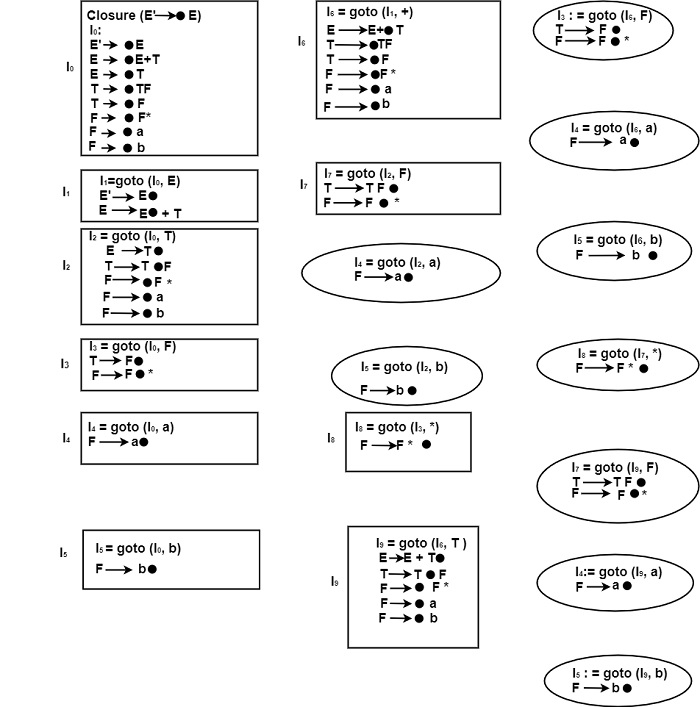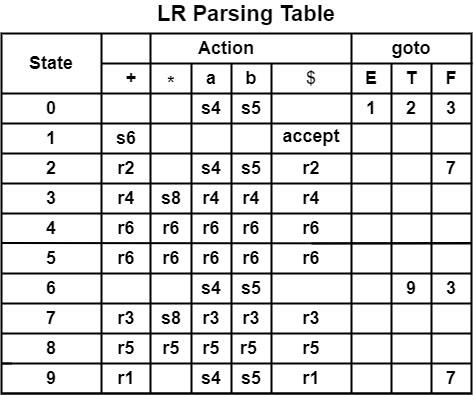# Construct the SLR Parsing table for the following grammar. Also, Parse the input string a * b + a.

E → E + T|T

T → TF|F

F → F*|a|b.

## Solution

Step1 − Construct the augmented grammar and number the productions.

(0) E′ → E

(1) E → E + T

(2) E → T

(3) T → TF

(4) T → F

(5) F → F ∗

(6) F → a

(7) F → b.

Step2 − Find closure & goto Functions to construct LR (0) items.

Box represents the New states, and the circle represents the Repeating State.Computation of FOLLOW

We can find out

FOLLOW(E) = {+, $} FOLLOW(T) = {+, a, b,$}

FOLLOW(F) = {+,*, a, b, $}Parsing for Input String a * b + a − Stack Input String Action 0 a * b + a$
Shift
0 a 4
* b + a $Reduce by F → a. 0 F 3 * b + a$
Shift
0 F 3 * 8
b + a $Reduce by F → F ∗ 0 F 3 b + a$
Reduce by T → F
0 T 2
b + a $Shift 0 T 2 b 5 +a$
Reduce by F → b
0 T 2 F 7
+a $Reduce by T → TF 0 T 2 +a$
Reduce by E → T
0 E 1
+a $Shift 0 E 1 + 6 a$
Shift
0 E 1 + 6 a 4
$Reduce by F → a 0 E 1 + 6 F 3$
Reduce by T → F
0 E 1 + 6 T 9
$Reduce by E → E + T 0 E 1$
Accept